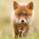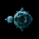2542 visningar
2542
Update:
added optional option for mapping the wicks to the high/low series(request for DCC ).
```study(title="[RS]RSI Divergence Candles V1")
useHL = input(title='Use high/low series for mapping the wicks?', type=bool, defval=false)
src = input(title='Source Series:', type=source, defval=close)
fast_length = input(title='Fast Length:', type=integer, defval=8)
slow_length = input(title='Slow Length:', type=integer, defval=55)
smooth = input(title='Smooth:', type=integer, defval=10)
overbought = input(title='Overbought Level:', type=float, defval=70)
oversold = input(title='Oversold Level:', type=float, defval=30)

fast_rsi = rsi(src, fast_length)
slow_rsi = rsi(src, slow_length)

smooth_fast_rsi = sma(fast_rsi, smooth)
smooth_slow_rsi = sma(slow_rsi, smooth)

rsi_high = useHL ? max(rsi(high, fast_length), rsi(high, slow_length)) : max(fast_rsi, slow_rsi)
rsi_low = useHL ? min(rsi(low, fast_length), rsi(low, slow_length)) : min(fast_rsi, slow_rsi)

plotcandle(smooth_slow_rsi, rsi_high, rsi_low, smooth_fast_rsi, title='rsi bars', color=smooth_fast_rsi>=smooth_slow_rsi?green:maroon)

hline(overbought, color=black)
hline(oversold, color=black)
```This one is good. Adding midline and a user option for candle color chart overlay option would be a help.
SvaraQuantitativeExhaustion
ill add midline to next version,about colors, currently its based on fast/slow smoothness, fast (over=green / under=maroon) slow.
Svarasmall difference....we can see the wicks hitting the outter limits of the RSI OB/OS levels where the original does not quite reach.
Svaracoondawg71
overlapped the RSI Divergence indicator with the RSI Candles by soruhttps://www.
Svara